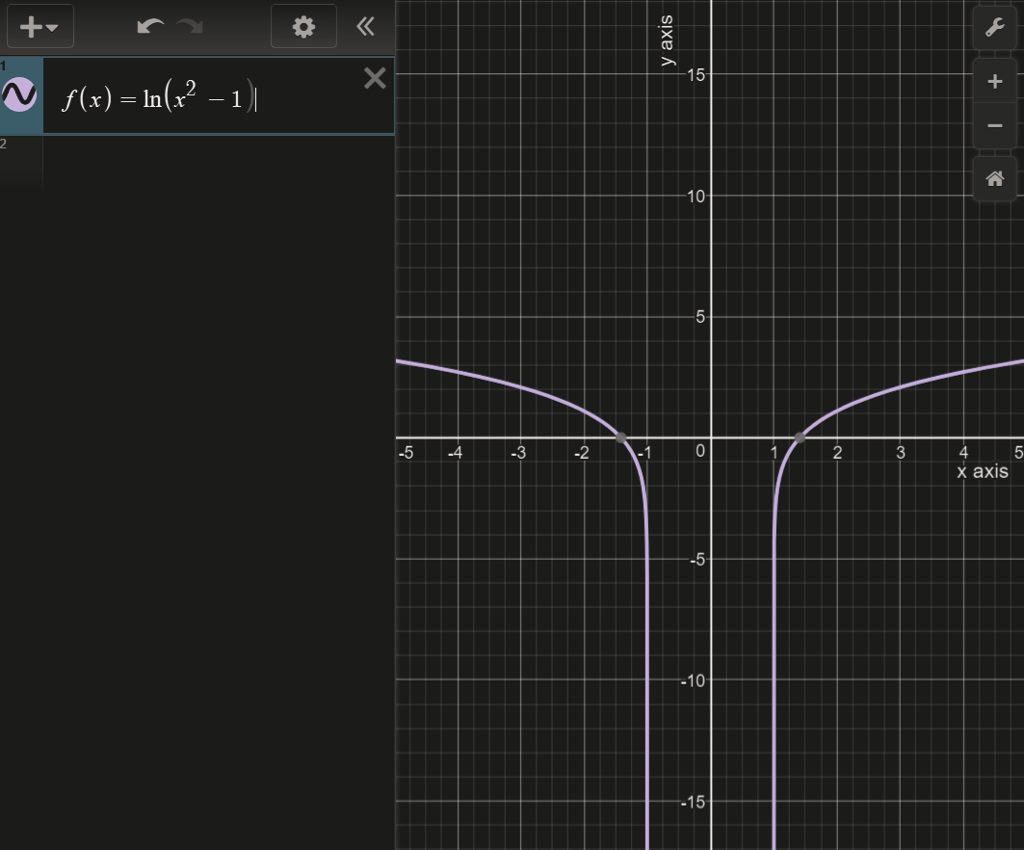# Find domain and range calculator

A graphing calculator is recommended. Find the domain and range of the function. (Enter your answers
using interval notation.) f(x) = ln(x2 − 1) f x x 2

domain

range

domain

range

domain

domain
range

range

given f(x) = ln(x2 ? 1)
function is defined when x2 ? 1>0
=>x2 > 1
=> x<-1 , x>1
domain is (-?,-1)U(1,?)
range is (-?,?)